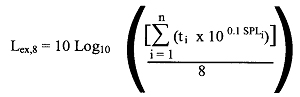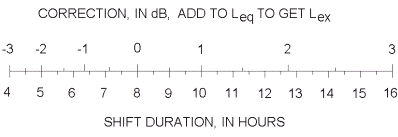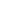# Appendix B: Determining Noise Exposure and Calculating Lex,8

• Issued: December 5, 2016
• Content last reviewed: December 2016

Disclaimer: This resource has been prepared to help the workplace parties understand some of their obligations under the Occupational Health and Safety Act (OHSA) and regulations. It is not legal advice. It is not intended to replace the OHSA or the regulations. For further information please see full disclaimer.

## Equivalent sound exposure level (Leq)

An “equivalent sound exposure level” (Leq) is the continuous sound pressure level (SPL) of noise averaged over time which contains the same amount of energy as that generated by the actual and varying sound levels being measured.

If a worker’s exposure to noise throughout a shift can be characterized by a number of Leq measurements for distinct noise activity periods, the measurements can be combined into a full shift Leq using the equation:

Leq, shift = 10 Log [(1/T) {(ti x 100.1SPLi ) + ……. .+ (tn x 100.1SPLn)}]

in which

Leq, shift is the full shift equivalent sound pressure level

T is the shift length in hours and equal to sum total of all activities from i=1 to i=n

i is a discrete activity of a worker exposed to a sound level

ti is the duration in hours of i,

n is the total number of discrete activities in the worker’s shift.

SPLi is the Leq for the ith activity in dBA

## Calculation of Lex,8

Lex,8” is the equivalent sound exposure level (Leq) of noise averaged over 8 hours, the length of a typical work shift. It can be calculated using the equation above, where T is equal to 8, or by the mathematical equation or formula given in the Noise Regulation (O. Reg. 381/15) below.in which,

Lex,8 is the equivalent sound exposure level in 8 hours,

∑ is the sum of the values in the enclosed expression for all activities from i = 1 to i = n,

i is a discrete activity of a worker exposed to a sound level,

ti  is the duration in hours of i,

SPLi is the sound level of i in dBA,

n is is the total number of discrete activities in the worker’s total workday.

For example, if a worker’s exposure consists of two distinct exposure periods, 3 hours at an Leq of 84 dBA and 5 hours at an Leq of 88 dBA, then:

Lex,8 = Leq, 8 = 10 Log [(1/8){(3 x 100.1 x 84) + (5 x 100.1 x 88)}] = 86.9 dBA

## Determination of Lex,8 for Non-Standard Work Shifts

### Method 1

For a shift length greater or less than 8 hours, the Lex,8 may be calculated using the equation:

Lex,8= Leq, T +10 Log (T/8), where T is the shift duration in hours.

For example, an Leq for a 10 hour shift would be converted to a Lex,8 as follows:

Lex,8= Leq, 10 + 10 Log (10/8) = Leq, 10 + 1 dBA

Therefore, if a worker is exposed to a measured level of 88 dBA Leq for a period of 10 hours, the Lex,8 would equal 88 dBA + 1 dBA, or 89 dBA.

### Method 2

The same result can be obtained using the following chart and table, which show the correction in dBA to be made to the Leq for different shift durations.In this chart the numbers below the line represent the actual shift duration in hours and the numbers above the line are the corresponding correction factor to be added to the shift Leq to convert it to an Lex,8.

 Correction (dB) Shift duration (hours) -3 -2 -1.3 -0.6 0 0.5 1 1.4 1.7 2 2.4 2.7 3 4 5 6 7 8 9 10 11 12 13 14 15 16

This table is provided for convenience only. It does not provide for the determinations of shifts or exposures for fractions of hours, such as shifts of 7.5 hours.

For a 12-hour shift, both the chart and the table show a 1.7 dBA correction (or more appropriately, a 2 dBA correction since measurement accuracy of better than +/-1 dBA is unlikely). Thus a worker exposed to 83 dBA for 12-hours would have an Lex,8 exposure of 83 + 2 = 85 dBA. Another way of looking at this is to consider the 12-hour shift Leq (or Lex, 12) limit to be 83 dBA.

### Method 3

Where a worker’s noise exposure can be easily split into discrete activities during which sound levels are steady, the following table may be used to determine the Leq for the shift.

Table of Equivalent Noise Exposures
82 16 hours
85 8 hours
88 4 hours
91 2 hours
94 1 hours
97 30 minutes
100 15 minutes
103 7.5 minutes
106 3.75 minutes
109 1.88 minutes
112 0.94 minutes
115 28.12 seconds

Where a worker is exposed to a steady sound level throughout their shift, the exposure duration must not exceed the value for that sound level in the above Table. Once the worker has reached the permissible duration for that sound level, the worker’s noise exposure has reached 100% of the permissible daily noise dose. For example, if a worker is exposed to a steady sound level of 91 dBA, the maximum permissible daily exposure duration is 2 hours.

Where the daily noise exposure is composed of two or more periods of noise exposure of different levels, the following equation may be used to determine if the overall exposure exceeds the allowable noise exposure limits:

(C1/T1 + C2/T2 + …. Cn/Tn) x 100 = % of exposure limit

Where

C = total duration of exposure at a specific noise level, and

T = total duration of exposure permitted at that level (Refer to “Table of Equivalent Noise Exposures” above)

The 85 dBA Lex,8 exposure limit is exceeded when the dose, calculated using the above formula exceeds 100%.

Example:

A worker is exposed to 85 dBA for 4 hours and 91 dBA for 1.5 hours.

Therefore using above calculation:

(4/8 + 1.5/2) x 100 = 125%

In this case the worker has exceeded his/her allowable noise exposure and therefore measures must be implemented to protect the worker’s hearing. If the exposure duration at 91 dBA were reduced to 1 hour, the combined exposure would be at 100% of the permissible daily noise dose, provided the worker’s exposure is at or below 80 dBA for the rest of his/her shift.

ISBN 978-1-4606-9207-3 (HTML)

## Follow usDisclaimer: This web resource has been prepared to assist the workplace parties in understanding some of their obligations under the Occupational Health and Safety Act (OHSA) and the regulations. It is not intended to replace the OHSA or the regulations and reference should always be made to the official version of the legislation.

It is the responsibility of the workplace parties to ensure compliance with the legislation. This web resource does not constitute legal advice. If you require assistance with respect to the interpretation of the legislation and its potential application in specific circumstances, please contact your legal counsel.

While this web resource will also be available to Ministry of Labour inspectors, they will apply and enforce the OHSA and its regulations based on the facts as they may find them in the workplace. This web resource does not affect their enforcement discretion in any way.